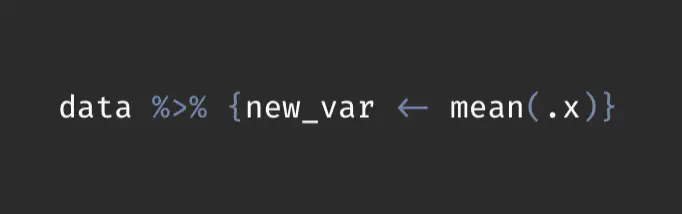# Creating Local Variables Within Pipe ChainsA few months ago, I discovered that it is possible to create a local variable within a tidyverse pipe chain. For example: `data %>% {new_var <- mean(.,x)}`

To me, this was an irrationally exciting discovery! Why? If you’re like me and you much prefer having a single pipe chain versus several separated functions and stored objects in your environment, the ability to create local variables on the fly within a pipe chain results in some incredibly efficient (and at least to me, pleasing) code.

To provide an example, let’s say that we want to create a table of means for some variables, and within the column headers, we want to add another statistic about the columns within the label.

Let’s use the trusty iris data set.

``library(tidyverse)``
``## ── Attaching packages ─────────────────────────────────────── tidyverse 1.3.1 ──``
``````## ✔ ggplot2 3.3.6     ✔ purrr   0.3.4
## ✔ tibble  3.1.8     ✔ dplyr   1.0.9
## ✔ tidyr   1.2.0     ✔ stringr 1.4.0
## ✔ readr   2.1.2     ✔ forcats 0.5.1``````
``````## ── Conflicts ────────────────────────────────────────── tidyverse_conflicts() ──
``````data <- iris %>%
janitor::clean_names()

``````##   sepal_length sepal_width petal_length petal_width species
## 1          5.1         3.5          1.4         0.2  setosa
## 2          4.9         3.0          1.4         0.2  setosa
## 3          4.7         3.2          1.3         0.2  setosa
## 4          4.6         3.1          1.5         0.2  setosa
## 5          5.0         3.6          1.4         0.2  setosa
## 6          5.4         3.9          1.7         0.4  setosa``````

If you’re unfamiliar with the iris data, there are four species of iris included, with four attribute variables describing the plants. Which attribute contains the most variation across the different species? One reasonable way to answer this question would be to 1) find the means of each attribute across the species, and then 2) calculate the standard deviation of the means across each attribute. Let’s make a table containing all of this information.

# Creating table

First I need to calculate the means for each variable.

``````data %>%
group_by(species) %>%
summarize(
across(everything(), ~ mean(.x))
)``````
``````## # A tibble: 3 × 5
##   species    sepal_length sepal_width petal_length petal_width
##   <fct>             <dbl>       <dbl>        <dbl>       <dbl>
## 1 setosa             5.01        3.43         1.46       0.246
## 2 versicolor         5.94        2.77         4.26       1.33
## 3 virginica          6.59        2.97         5.55       2.03``````

Easy enough. Now, the tricky part: How can we include information about the standard deviations of these columns? The first thought would be to calculate these values and somehow append them as a separate row. This would work fine, but it would be a bit difficult to format successfully. An alternative approach is to include the standard deviations within the column labels themselves. Normally this would involve a few clunky steps, but with the ability to create local variables within a pipe, this turns out to be a pretty easy and concise task!

``````data %>%
group_by(species) %>%
summarize(
across(everything(), ~ mean(.x))
) %>%
{
sds <- summarize(.,across(-1, ~ sd(.x))) %>% # calculating SDs of each column which are stored in a local variable, "sds".
mutate(across(everything(), ~ round(.x,2))) # rounding SDs

rename_with(., .cols = -1, ~ paste0(.x," (SD = ",sds[1,],")")) #renaming columns to include SD values
} %>%
gt::gt()``````
species sepal_length (SD = 0.8) sepal_width (SD = 0.34) petal_length (SD = 2.09) petal_width (SD = 0.9)
setosa 5.006 3.428 1.462 0.246
versicolor 5.936 2.770 4.260 1.326
virginica 6.588 2.974 5.552 2.026

Pretty cool, huh? But what if you wanted to store the `sds` object for something later on? Easy: assign it to a global variable!

``````data %>%
group_by(species) %>%
summarize(
across(everything(), ~ mean(.x))
) %>%
{
sds <- summarize(., across(-1, ~ sd(.x))) %>% # calculating SDs of each column which are stored in a local variable, "sds".
mutate(across(everything(), ~ round(.x,2))) # rounding SDs

SDs <<- sds # Creating global variable which will be saved in environment

rename_with(., .cols = -1, ~ paste0(.x," (SD = ",sds[1,],")")) #renaming columns to include SD values
} %>%
gt::gt()``````
species sepal_length (SD = 0.8) sepal_width (SD = 0.34) petal_length (SD = 2.09) petal_width (SD = 0.9)
setosa 5.006 3.428 1.462 0.246
versicolor 5.936 2.770 4.260 1.326
virginica 6.588 2.974 5.552 2.026

And here is the stored `SDs` object:

``SDs``
``````## # A tibble: 1 × 4
##   sepal_length sepal_width petal_length petal_width
##          <dbl>       <dbl>        <dbl>       <dbl>
## 1          0.8        0.34         2.09         0.9``````

There are plenty more useful applications beyond what I’ve shown here, but hopefully this example provides a glimpse into the potential power of using local variables within pipes.##### Sean Bock
###### PhD Candidate in Sociology

I am interested in Quantitative Research, Natural Language Processing, Survey Research, and Data Visualization